# Non-Global Privacy Protection Facing Sensitive Areas in Face Images

Non-Global Privacy Protection Facing Sensitive Areas in Face Images

Chao Liu Jing YangYining Zhang Xuan Zhang Weinan Zhao Fengjuan Miao Yukun Shao

College of Computer Science and Technology, Harbin Engineering University, Harbin 150001, China

College of Communication and Electronic Engineering, Qiaihar University, Qiqihar 161000, China

Department of Computer Engineering, DaQing Vocational College, Daqing 163000, China

Information Center, First Affiliated Hospital of Qiqihar Medical College, Qiqihar 161000, China

Corresponding Author Email:
yangjing@hrbeu.edu.cn
Page:
1677-1687
|
DOI:
https://doi.org/10.18280/ts.380611
8 July 2021
|
Accepted:
2 November 2021
|
Published:
31 December 2021
| Citation

OPEN ACCESS

Abstract:

Face images, as an information carrier, are naturally weak in privacy. If they are collected and analyzed by malicious third parties, personal privacy will leak, and many other unmeasurable losses will occur. Differential privacy protection of face images is mainly being studied under non-interactive frameworks. However, the ε-effect impacts the entire image under these frameworks. Besides, the noise influence is uniform across the protected image, during the realization of the Laplace mechanism. The differential privacy of face images under interactive mechanisms can protect the privacy of different areas to different degrees, but the total error is still constrained by the image size. To solve the problem, this paper proposes a non-global privacy protection method for sensitive areas in face images, known as differential privacy of landmark positioning (DPLP). The proposed algorithm is realized as follows: Firstly, the active shape model (ASM) algorithm was adopted to position the area of each face landmark. If the landmark overlaps a subgraph of the original image, then the subgraph would be taken as a sensitive area. Then, the sensitive area was treated as the seed for regional growth, following the fusion similarity measurement mechanism (FSMM). In our method, the privacy budget is only allocated to the seed; whether any other insensitive area would be protected depends on whether the area exists in a growing region. In addition, when a subgraph meets the criterion for merging with multiple seeds, the most reasonable seed to be merged would be selected by the exponential mechanism. Experimental results show that the DPLP algorithm satisfies ε-differential privacy, its total error does not change with image size, and the noisy image remains highly available.

Keywords:

differential privacy, interactive framework, non-globality, landmark positioning, regional growth

1. Introduction

The rapid advancement of information technology makes it easier to acquire and share face images. People can easily obtain others’ photos via online social networks, mobile payment, surveillance systems, and other channels. These digital images are rich in sensitive personal information. If they are collected and analyzed by malicious third parties, personal privacy will leak, and many other unmeasurable losses will occur . Relevant stakeholders have expressed their concerns over the possible leak of personal privacy during the use of face images: a person may be kept in the dark about the public recognition and tracking of him/her, and about the collection, use, and sharing his/her personal data. To make matters worse, face image databases could be sold or shared by many parties to unknown buyers or sharers.

The image data query under privacy protection provides a solution to the above-mentioned privacy problems. For example, Fung et al.  and Xiao and Tao  created the k-same method, using the anonymization mechanism. However, there is a major weakness of the conventional anonymization mechanism: Prior to processing the data, it is necessary to set many prior conditions about the background knowledge and attack models of the attacker, many of which does not hold in the real world. For instance, Li et al.  restricted user access to images on social networks through access control. Instead of protecting image privacy, their strategy protects images from the angle of access setting. Terrovitis et al.  implemented same-state encryption of gray images. But data encryption still needs to make assumptions of the attacks. Focusing on anonymous images uploaded to Facebook, Sweeney  found that attackers can derive the social security number (SSN) of those in the anonymous images from Friendster, an extra feature of this social network.

Differential privacy , proposed by Dwork in 2006, adds noise to the output to disturb sensitive data. Under differential privacy, the effect of a single record can be concealed. Whether he record is in the dataset or not, the same result will always be outputted by the same probability, which prevents the attacker from further reasoning. Dwork further studied differential privacy in a series of papers [8-12], and disclosed the realization mechanism of differential privacy [13, 14]. McSherry noted that sequence combination and concurrent combination must be satisfied by the differential privacy algorithm for complex privacy problems .

The data protected by differential privacy can be published interactively or non-interactively, depending on the realization environment. The following are some representative interactive data publication methods: Roth and Roughgarden  Median algorithm, which can respond to multiple queries; Hardt and Rothblum  private multiplicative weights (PMW) mechanism, which increases the number of queries; Gupta et al.  universal iterative dataset creation (IDC) framework; Fan and Xiong  filtering and adaptive sampling for releasing time series under differential privacy (FAST) algorithm; Kellaris et al.  flow data publication algorithm with an unlimited number of queries. Non-interactive data publication mainly uses histogram publication. The following are some representative non-interactive data publication methods: Xiao et al.  Privelet algorithm; Xu et al.  Noise First and Structure First algorithms; Li et al.  matrix mechanism; Li et al.  data- and workload-aware (DAWA) algorithm. Under the framework of differential privacy, the sensitive information in the protected images has only been researched tentatively, because the image data are highly complex. Currently, the additive noise for the differential privacy protection of images impacts the entire image. For face images, the sensitive information of the face only concentrates in the face area, and even in some specific parts of the face area. The other insensitive areas have nothing to do with privacy leak of face images.

Through the above analysis, this paper puts forward the differential privacy of landmark positioning (DPLP) algorithm, a non-global privacy protection method for sensitive areas in face images, details the realization of the algorithm, and experimentally verifies the performance of the DPLP in protecting the privacy of face images.

2. Preliminaries

2.1 Differential privacy

Definition 1. Adjacent datasets of face image

For a given image X, the gray matrix Xmn can be obtained by normalizing the image. Then, there exists $X|X_{mn}=\left[\begin{array}{ccc}\mathrm{X}_{11}, \mathrm{X}_{12}, & \cdots & \mathrm{X}_{1 \mathrm{n}} \\ \vdots & \ddots & \vdots \\ \mathrm{X}_{\mathrm{m} 1}, \mathrm{X}_{\mathrm{m} 1}, & \cdots & \mathrm{X}_{\mathrm{mn}}\end{array}\right]$, where xij in matrix Xmn represents the gray of the corresponding element. If there exists an X' with only one element difference from X, $\left|X-X^{\prime}\right|=x_{i j}(1 \leq i \leq m, 1 \leq j \leq n)$, then X and X' are adjacent datasets.

Definition 2. Differential privacy

For a given random algorithm M of image data publication, with the output range of Range(M), the algorithm can provide ε- differential privacy, if its arbitrary outputs on two adjacent gray images X and X' satisfy:

$\operatorname{Pr}[\mathrm{M}(\mathrm{X}) \in \mathrm{S}] \leq \exp (\varepsilon) \times \operatorname{Pr}\left[\mathrm{M}\left(\mathrm{X}^{\prime}\right) \in \mathrm{S}\right]$                 (1)

Definition 3. Global sensitivity

Let Q be a random query function meeting $Q: D \rightarrow R^{n}$. Then, the global sensitivity of Q can be expressed as:

$\Delta Q_{G S}=\max _{X, X^{\prime}}\left\|Q(D)-Q\left(D^{\prime}\right)\right\|_{\rho}$                      (2)

Theorem 1. Laplace mechanism

Let Q be a query series of the length d. The random algorithm M receives database D and outputs the following vector that satisfy ε-differential privacy:

$\left.M(D)=Q(D)+<\operatorname{Lap}_{1}\left(\frac{\Delta Q_{L S}}{\varepsilon}\right), \ldots, \operatorname{Lap}_{d}\left(\frac{\Delta Q_{L S}}{\varepsilon}\right)\right)>$            (3)

Theorem 2. Exponential mechanism

The exponential mechanism mainly handles the non-numeric outputs of the sampling algorithm. Under any exponential mechanism, the sampling algorithm M satisfies ε-differential privacy if it meets:

$M(X, u)=\left\{r: \mid \operatorname{Pr}[r \in S] \propto \exp \left(\frac{\varepsilon u(X, r)}{2 \Delta u_{I . S}}\right)\right\}$                   (4)

where, u(X,r) is the scoring function; $\Delta \mathrm{u}_{\mathrm{LS}}$ is the global sensitivity of the scoring function u(X,r); S is the output domain of our algorithm; r is the selected output term of the output domain S. The higher the score of u(X,r), the greater the probability for r being selected as the output.

Property 1. Differential privacy-serial combination property

For a given dataset X and a set of differential privacy algorithms $\mathrm{M}_{1}(\mathrm{X}), \mathrm{M}_{2}(\mathrm{X}), \ldots, \mathrm{M}_{\mathrm{m}}(\mathrm{X})$ related to X, if algorithm Mi(D) satisfies εi- differential privacy, and the random processes of any two algorithms are independent of each other, then the algorithm combined from these algorithms satisfies $\sum_{\mathrm{i}=1}^{\mathrm{m}} \varepsilon_{\mathrm{i}}$- differential privacy.

Property 2. Differential privacy-parallel combination property

Let $\mathrm{M}_{1}\left(\mathrm{X}_{1}\right), \mathrm{M}_{2}\left(\mathrm{X}_{2}\right), \ldots, \mathrm{M}_{\mathrm{m}}\left(\mathrm{X}_{\mathrm{m}}\right)$ be a series of ε-differential privacy algorithms with input datasets $X_{1}, X_{2}, \ldots, X_{m}$, respectively. Suppose the random processes of any two algorithms are independent of each other. Then, the algorithm combined from these algorithms satisfies ε-differential privacy.

2.2 Face landmark positioning

Face landmark positioning can automatically detect the landmarks (positions) in representative sensitive areas (eyebrows, eyes, ears, noise, mouth, and face contours) in face-containing images, using some algorithms. This technique is of great significance to research fields like face identification, expression analysis, three-dimensional (3D) face modeling, face synthesis, and face image coding. Yang and Huang  realized the mosaic algorithm with the aid of edge tracking. Meng et al.  were the first to position face landmarks through geometric projection. On this basis, Feng and Zhou developed face landmark positioning using variance projection function (VPF)  and integral projection function (IPF) , respectively. Zhang and Lenders  introduced binarization to position landmarks in reference to pupil positions. Considering the symmetry of face, Reisfeld et al.  proposed the generalized symmetric transform (GST), which takes the eyes as center points, to position face landmarks. Wu et al.  established multiple Snake models for different face organs, and fitted the locally converging Snake models into a complete set of face landmarks. Yuile et al.  replaced the traditional edge prediction algorithm with a more stable approach, namely, the peak-valley variation frequency of face image gray value, and put forward a face landmark positioning method based on deformable template (DT). Lanitis et al.  suggested using principal distribution model (PDM) algorithm to realize face landmark positioning. Cootes et al.  designed the active shape model (ASM) algorithm, a statistical point distribution model. Dollár et al.  positioned landmarks in images through cascaded pose regression (CPR). With the development of convolutional neural network (CNN) , many scholars utilized the strong feature extraction power of the CNN to pinpoint face landmarks. The representative methods include deep CNN (DCNN) , tasks-constrained deep convolutional network (TCDCN) , multitask cascaded convolutional networks (MTCNN) , and tweaked CNN (TCNN) . In addition, some scholars solved face landmark positioning with principal component analysis (PCA) , support vector machine (SVM) , back propagation network (BPN) , dynamic link architecture (DLA) , and Gabor wavelet network (GWN) . Table 1 analyzes the performance of the above methods.

Table 1. Performance of different face landmark positioning methods

 Algorithm Image quality Computational complexity Accuracy Mosaic image Strongly high Strongly complex Strongly low VPF Strongly high Slightly simple Slightly low IPF Strongly high Slightly simple Slightly low Binarization Strongly high Slightly simple Slightly low GST Strongly high Strongly complex Slightly high Snake Strongly high Strongly complex Slightly high DT Strongly high Slightly complex Slightly high ASM Slightly high Slightly complex Strongly high CPR Slightly high Strongly complex Strongly high DCNN Strongly low Strongly complex Strongly high TCDCN Strongly low Strongly complex Strongly high MTCNN Strongly low Strongly complex Strongly high TCNN Strongly low Strongly complex Strongly high PCA Strongly low Strongly complex Strongly high SVM Strongly low Strongly complex Strongly high BPN Slightly low Slightly complex Strongly high DLA Slightly low Slightly complex Strongly high GWN Slightly low Slightly complex Strongly high
3. Methodology

3.1 Laplacian (LAP) algorithm

This paper proposes the LAP algorithm based on the Laplace mechanism. Without changing any of the original data, this algorithm directly disturbs the two-dimensional (2D) matrix generated from the original image with Laplace noise, and publishes the disturbed image straightforwardly. In the LAP algorithm, every pixel xij in the gray matrix Xmxn is considered an independent individual, laying the basis for applying the interactive mechanism to privacy protection. Provided that the pixels do not disturb each other, and the privacy budget is allocated evenly, each xij(1≤i≤m, 1≤j≤n) consumes a privacy budget of ε/(mxn). The LAP algorithm is detailed as follows:

Different from the original image X, the privacy protected image X' contains an additive noise of $2 \mathrm{~m} \times \mathrm{n} \times(\Delta \mathrm{Q} \times \mathrm{m} \times \mathrm{n} / \varepsilon)^{2}$. Although the LAP algorithm satisfies ε-differential privacy, a huge error will arise if the algorithm is directly applied for privacy protection of an excessively large image, making the noisy image strongly unavailable. The LAP algorithm applies to noise addition to the subgraphs of an image, but the noise level of a subgraph depends on the size of that subgraph.

Most of the existing methods face the same problem as the LAP algorithm: the algorithm feasibility is limited by image size. Besides, there are two more defects with these methods: an excessively high computing load, and the low availability of the noisy image.

 Algorithm 1: LAP Input: original image X, privacy budget ε, parameters m and n, subgraph similarity expectation Th Output: Image X' satisfying differential privacy Read the original image X, convert the image into gray matrix and store it in matrix Xmxn for i=1 to m for i=1 to n $X_{(i, j)}{ }^{\prime}=X_{(j, i)}+\operatorname{lap}(\Delta Q \times m \times n / \varepsilon)$ end for end for Output privacy protected image X'

3.2 ASM algorithm

The ASM model abstracts the target by a shape model. It is an algorithm based on point distribution model (PDM). In the PDM, the geometry of objects with similar shapes, such as face, hands, heart, and lungs, can be described by a shape vector, which is formed by serial connected coordinates of several landmarks. Before implementing the ASM algorithm, it is necessary to manually label the training images, obtain the shape model through training, match specific objects with landmarks. The ASM algorithm is realized in two stages: training and search.

During ASM training, the first step is to establish a shape model that matches any face shapes. The model construction requires the collection of sufficient training samples, and manual labeling of face landmarks (In this paper, 68 landmarks are labeled on each training image). After labeling, all landmarks are combined into a shape vector $g_{i}=\left(x_{1}^{i}, y_{1}^{i}, x_{2}^{i}, y_{2}^{i}, \ldots, x_{k}^{i}, y_{k}^{i}\right)(i=1,2, \ldots, n)$, where $k=68$, and $\left(x_{j}^{i}, y_{j}^{i}\right)$ is the position of landmark j on training sample i, and n depends on the number of training samples. In this way, a 2xn-dimensional vector a can be obtained:

$g_{i}=\left(x_{1}, \ldots x_{n}, y_{1} \ldots y_{n}\right)^{T}$               (5)

The high dimensionality of vector (5) is not conducive to the subsequent computing. But the different dimensions have a strong correlation, owing to the natural similarity of face features, even if the landmarks come from different faces. However, there is no excessively large position changes between the landmarks with the same label. To solve the excessively high dimensionality, the vector is subjected to dimensionality reduction and principal component extraction by the PCA. Hence, any set of landmarks can be viewed as a point in the principal component space. The mean of the point set is the origin. Thus, any point can be described as the sum of the origin and a vector. Thus, we have:

$g_{i} \approx \bar{g}+P b$                 (6)

During the PCA, the mean shape vector needs to be calculated:

$\bar{g}=\frac{1}{n} \sum_{i=1}^{n} g_{i}$                (7)

Then, the covariance matrix can be derived:

$S=\frac{1}{n} \sum_{i=1}^{n}\left(g_{i}-\bar{g}\right)^{T} \cdot\left(g_{i}-\bar{g}\right)$                  (8)

After that, the eigenvalues of the covariance matrix S are calculated, and sorted to obtain $\tau_{1}, \tau_{2}, \ldots \tau_{q,}$, where any $\tau_{i}>0$. The P in formula (6) is a covariance matrix containing the top-t principal components. The matrix P and the corresponding eigenvalue satisfy:

$\frac{\sum_{i=1}^{t} \tau_{i}}{\sum_{i=1}^{q} \tau_{s}}>f_{v} V_{T}$                   (9)

where, fv is a proportionality coefficient depending on the number of eigenvectors (the value of fv is usually 95%); VT is the sum of all eigenvalues. The b in formula (6) is a t-dimensional vector controlling the variation in landmark shape:

$b_{i}=P^{T} \cdot\left(g_{i}-\bar{g}\right)$                   (10)

when b=0, x is the origin (mean shape). To prevent the shape variation from exceeding the preset range, the b value should be limited by:

$D_{m}^{2}=\sum_{i=1}^{t}\left(\frac{b_{i}{ }^{2}}{\tau_{i}}\right) \leq D_{\max }^{2}$                     (11)

where, Dmax is usually set to 3. Thus, $\left|b_{i}\right|<3 \sqrt{\tau_{i}}$.

After setting up the shape model, it is necessary to establish the local features of each landmark, such as to match the shape model with the new point set. For a given face image X, the image matching process aims to rotate, scale, and translate the shape model:

$X=T_{X_{t}, Y_{t}, s, \theta}(\bar{g}+P b)$                  (12)

where, $T_{X_{t}, Y_{t}, s, \theta}$ is the matching operation matrix:

$T_{X_{t}, Y_{t}, s, \theta}\left(\begin{array}{l}X \\ y\end{array}\right)=\left(\begin{array}{l}X_{t} \\ Y_{t}\end{array}\right)+\left(\begin{array}{ll}s \cos \theta & -s \sin \theta \\ s \sin \theta & s \cos \theta\end{array}\right)$                   (13)

To match the landmark set Y (the results after translation, scaling, and rotation) and model X, i.e., minimize the difference between model X and image landmark set Y, it is necessary to solve the minimum of the following formula:

$\left|Y-T_{X_{t}, Y_{t}, s, \theta}(\bar{g}+P b)\right|^{2}$                  (14)

Note that, if the model point positions are given in X, and if the nearest adjacent point to each model point is X’, then the error metric can be expressed as:

$F\left(b, X_{t}, Y_{t}, s, \theta\right)=\left|X-X^{\prime}\right|^{2}$                    (15)

During ASM search, the mean shape needs to go through affine transformation to obtain an initial model:

$X=M(s, \theta)\left[g_{i}\right]+X_{c}$                   (16)

The initial model X (16) refers to the results of the mean shape after being rotated counterclockwise by $\theta$ degrees, scaled by a ratio of s, and translated by a distance of Xc. Using the initial model, the search stage looks for the target shape in image Y, aiming to minimize the gap between the landmark position of the target shape and the actual landmark position. The final results of the search are obtained by adjusting the variation of parameter b and implementing affine transformation. The specific steps of the ASM search are explained in Algorithm 2.

 Algorithm 2: ASM search L=Lmax while ($L \geq 0$)    Calculate the position of the model point in the L-th layer of the image      Search n sampling points on both sides of the model point      Update the position and adjust the parameters to fit the model to the new point      if (the preset number of iterations is exceeded or no closer feature point is found at the current position)          Return to line 2      if (L>1) then $\mathrm{L} \rightarrow(\mathrm{L}-1)$ if (convergence of layer 0 function)      Output result

3.3 DPLP algorithm

Non-interactive data publication is the research hotspot of the data publication under differential privacy. In this form of data publication, the entire image is influenced by ε-effect. As a result, the noise level obeys uniform distribution across the image. However, the sensitive information only exists in specific areas of real-world face images. For an image, different parts require different degrees of privacy protection. Thus, it is meaningful to realize non-global privacy protection of human images, and reduce the noise impact on the protected image. This paper combines landmark positioning, regional growth, and differential privacy into a novel face image privacy protection method called DPLP. Our algorithm adopts the ASM algorithm to estimate the areas of sensitive information, and implements non-global noise disturbance to the specific areas containing sensitive face information. Under the premise of satisfying ε-differential privacy, the DPLP algorithm mitigates the influence of Laplace noise on the privacy protected image, and balances image availability and the degree of privacy protection.

To ensure the realization of the DPLP algorithm, four key problems must be solved during the algorithm design: selecting landmark positioning algorithm, setting the rules for regional growth, allocation of privacy budget, and selecting the best target out of multiple seeds available for a subgraph.

The setting of the rules for regional growth is crucial to regional growth. Due to the limitations of the traditional regional growth rules, this paper adopts the fusion similarity measurement mechanism (FSMM)  as regional growth rules. The FSMM(X,Y)  can be calculated by:

$\operatorname{FSMM}(X, Y)=\frac{\quad \begin{array}{c}\varphi \times(d(X, Y) / \rho(X, Y)+\sigma) \\ \times\left(2 u_{X} u_{Y}+C_{1}\right) \times\left(2 \sigma_{X} \sigma_{Y}+C_{2}\right)\end{array}}{\left(u_{X}^{2}+u_{Y}^{2}+C_{1}\right) \times\left(\sigma_{X}^{2}+\sigma_{Y}^{2}+C_{2}\right)}$                      (17)

The regional growth of the DPLP algorithm needs to allocate a suitable portion of the privacy budget to each seed, using the Laplace mechanism of differential privacy. Whether an adjacent subgraph should be merged into the growing region of the current seed depends on the similarity between the subgraph and the region meets the preset threshold. In our method, the privacy budget is only allocated among the seeds. For an insensitive area, if it belongs to a growth region, it will be protected; otherwise, it will not be protected. In addition, when a subgraph can be merged with multiple seed areas, the most suitable seed area to be merged needs to be selected through the exponential mechanism. The realization of the DPLP algorithm is as follows:

Lines 1-5 convert the original image into a gray image, divide the gray image into β subgraphs of the same size, and call the ASM algorithm to position 68 landmarks and to find the seeds needed for regional growth. Line 5 adjusts the position of seed regions to ensure the growth direction. Line 6 divides the total privacy budget ε into two parts: the part ε1 for adding Laplace noise to seed regions, and the part ε2 for implementing the exponential mechanism. Line 7 defines the possibility of $\leq S$: a subgraph may cover multiple landmarks, because the subgraph size is too large. Lines 8-20 overcome the defect of traditional regional growth technique: the adjacent subgraph can be merged to the growth region of the current seed, as long as the subgraph satisfies the growth rules; when multiple seed areas are available for merging the subgraph, the traditional technique depends both on growth rules and the sequence of seed selection. To solve the problem, Lines 15-16 take the Euclidean distance as the scoring function between each subgraph and each seed, and choose the most suitable seed by the exponential mechanism. The nearest seed is not preferred for region fusion. Otherwise, the attacker can deduce the distribution of landmarks, a prediction result of face identification, leading to privacy leak.

 Algorithm 3: DPLP Input: original image X, privacy budget ε, preset parameters β, subgraph similarity expectation Th Output: image X' satisfying differential privacy Read the original image X, convert the image into gray matrix and store it in matrix Xmxn Xmxn according to preset parameters β, split into subgraph sets with the same structure T(I,J) Extracting facial feature point $K_{S}=\left(K_{1}, K_{2}, \ldots K_{68}\right)$ using ASM algorithm_ S If KS belongs to a subgraph $T_{(i, j)}(1 \leq \mathrm{i} \leq I, 1 \leq \mathrm{j} \leq J)$ Set T(i,j) as the backup seed, optimize the position of T(i,j), and add it to the SeedN, $\varepsilon=\varepsilon_{1}+\varepsilon_{2}$ Find all backup Seed $_{N}=\left(\right.$ Seed $_{1}$, Seed $_{2}, \ldots$ Seed $\left._{n}\right) \quad(N \leq S)$ $\widetilde{\text { Seed }}=0$ Create the list to record the status of the current seed region merging process while $(\widetilde{\text { Seed }} \leq N)$ if list_head $==N U L L$ Randomly select unmarked Seedn and add noise to it, $\operatorname{Seed}_{n}^{\prime}=\operatorname{Seed}_{n}+\operatorname{lap}\left(\Delta Q \times N / \varepsilon_{1}\right)$     If ($\operatorname{FSMM}\left(\operatorname{Seed}_{n}^{\prime}, T_{(i, j)}\right) \leq T h \& \& T_{(i, j)}$ is unmarked      Calculate all SeedM that meets the condition by $\operatorname{FSMM}\left(\right.$ Seed $\left._{N}^{\prime}, T_{(i, j)}\right) \leq$ Th $\left(\right.$ Seed $_{M} \in$ Seed $_{N} \mid$ Seed $_{M}=$$\left(\ldots\right.$, Seed $_{m} \mid$ Seed $\left._{m}=T_{(x, y)}, \ldots\right)$      $u\left(T_{(i, j)}\right.$, Seed $\left._{M}\right)=-\sqrt{(i-x)^{2}+(j-y)^{2}}$      Extract Seed $_{m} \mid$ Seed $_{m} \in$ Seed $_{M}\left(\mathrm{P} \propto \exp \left(\varepsilon_{2} u\left(T_{(i, j)}\right.\right.\right.$, Seed $\left.\left.\left._{M}\right) / 2 \Delta u\right)\right)$      $T_{(i, j)}^{\prime}=\operatorname{Seed}_{m}+\operatorname{lap}\left(\Delta Q \times N / \varepsilon_{1}\right)$      Seedn as marked, T(i,j) as marked      $\widetilde{\text { Seed }}=\widetilde{\text { Seed }}+1$      Release all data in the list, and the consolidation of this sub region is comple

Theorem 3. In DPLP, the privacy budget under the Laplace mechanism will not surpass ε1.

Proof: According to the constraint of $N \leq S$ in Line 7, Algorithm 3, when each seed only contains one landmark, N=(S=68). Then, there exists:

$\varepsilon_{1}^{1}+\varepsilon_{1}^{2}+\varepsilon_{1}^{3}+\cdots+\varepsilon_{1}^{68}=\varepsilon_{1}$

when each seed contains more landmarks, N=(S=68). Then, there exists:

$\varepsilon_{1}^{1}+\varepsilon_{1}^{2}+\varepsilon_{1}^{3}+\cdots+\varepsilon_{1}^{N}<\varepsilon_{1}$

Q.E.D.

Theorem 4. DPLP satisfies ε-differential privacy.

Proof:

In the DPLP, the total privacy budget ε is divided into two parts: $\varepsilon=\varepsilon_{1}+\varepsilon_{2}$. Among them, ε2 is used to select the target seed by the exponential mechanism. The selection probability is positively proportional to $exp\left(\frac{\varepsilon_{2} u\left(T_{(i, j)}, \text { Seed }_{M}\right)}{2 \Delta u}\right)$. For convenience, the scoring function $u\left(T_{(i, j)}\right.$, Seed $\left._{M}\right)$ is replaced with $\Delta Q$, that is, there exists:

$\operatorname{Pr}\left[M\left(\operatorname{Seed}_{M}, \Delta Q\right)=\operatorname{Seed}_{m}\right]$

$=\frac{\exp \left(\frac{\varepsilon_{2} \Delta Q\left(\text { Seed }_{M}, \text { Seed }_{m}\right)}{2 \Delta u}\right)}{\sum_{\text {Seed }_{m}^{\prime} \in O} \exp \left(\frac{\varepsilon_{2} \Delta Q\left(\text { Seed }_{M}, \text { Seed }_{m}^{\prime}\right)}{2 \Delta u}\right)}$                   (18)

Given SeedM and its adjacent SeedM', for any Seedm value (Seed $_{m} \in O$), the following can be derived from formula (18):

$\frac{\operatorname{Pr}\left[M\left(\operatorname{Seed}_{M}, \Delta Q\right)=\operatorname{Seed}_{m}\right]}{\operatorname{Pr}\left[M\left(\operatorname{Seed}_{M}{ }^{\prime}, \Delta Q\right)=\operatorname{Seed}_{m}\right]}=\frac{\frac{\exp \left(\frac{\varepsilon_{2} \Delta Q\left(\text { Seed }_{M}, \text { Seed }_{m}\right)}{2 \Delta u}\right)}{\sum_{S e e d_{m}{ }^{\prime} \in O} \exp \left(\frac{\varepsilon_{2} \Delta Q\left(\text { Seed }_{M}, \text { Seed }_{m}{ }^{\prime}\right)}{2 \Delta u}\right)}}{\frac{\exp \left(\frac{\varepsilon_{2} \Delta Q\left(\text { Seed }_{M}{ }^{\prime}, \text { Seed }_{m}\right)}{2 \Delta u}\right)}{\sum_{\text {Seed }_{m}{ }^{\prime} \in O} \exp \left(\frac{\varepsilon_{2} \Delta Q\left(\text { Seed }_{M}{ }^{\prime}, \text { Seed }_{m}{ }^{\prime}\right)}{2 \Delta u}\right)}}$

$=\left(\frac{\exp \left(\frac{\varepsilon_{2} \Delta Q\left(\left(\text { Seed }_{M}, \text { Seed }_{m}\right)\right)}{2 \Delta u}\right)}{\exp \left(\frac{\varepsilon_{2} \Delta Q\left(\text { Seed }_{M}^{\prime}, \text { Seed }_{m}\right)}{2 \Delta u}\right)}\right) \times\left(\frac{\sum_{S e e d_{m}^{\prime} \in O} \exp \left(\frac{\varepsilon_{2} \Delta Q\left(\text { Seed }_{M}^{\prime}, \text { Seed }_{m}^{\prime}\right)}{2 \Delta u}\right)}{\sum_{S e e d_{m}^{\prime} \in O} \exp \left(\frac{\varepsilon_{2} \Delta Q\left(\text { Seed }_{M}, \text { Seed }_{m}^{\prime}\right)}{2 \Delta u}\right)}\right)$

$=\exp \left(\frac{\varepsilon_{2}\left(\begin{array}{c}\varepsilon_{2} \Delta Q\left(\text { Seed }_{M}, \text { Seed }_{m}\right) \\ -\varepsilon_{2} \Delta Q\left(\text { Seed }_{M}^{\prime}, \text { Seed }_{m}\right)\end{array}\right)}{2 \Delta u}\right) \times\left(\frac{\sum_{\text {Seed }_{m}^{\prime} \in O} \exp \left(\frac{\varepsilon_{2} \Delta Q\left(\text { Seed }_{M}^{\prime}, \text { Seed }_{m}^{\prime}\right)}{2 \Delta u}\right)}{\sum_{\text {Seed }_{m}^{\prime} \in O} \exp \left(\frac{\varepsilon_{2} \Delta Q\left(\text { Seed }_{M}, \text { Seed }_{m}^{\prime}\right)}{2 \Delta u}\right)}\right)$

$\leq \exp \left(\frac{\varepsilon_{2}}{2}\right) \times\left(\frac{\sum_{S e e d_{m}^{\prime} \in O} \exp \left(\frac{\varepsilon_{2}}{2}\right) \times \exp \left(\frac{\varepsilon_{2} \Delta Q\left(S e e d_{M}, S e e d_{m}^{\prime}\right)}{2 \Delta u}\right)}{\sum_{S e e d_{m}^{\prime} \in O} \exp \left(\frac{\varepsilon_{2} \Delta Q\left(S e e d_{M}, S e e d_{m}^{\prime}\right)}{2 \Delta u}\right)}\right)$

$\leq \exp \left(\frac{\varepsilon_{2}}{2}\right) \times \exp \left(\frac{\varepsilon_{2}}{2}\right) \times\left(\frac{\sum_{\text {Seed }_{m}{ }^{\prime} \in O} \exp \left(\frac{\varepsilon_{2} \Delta Q\left(\text { Seed }_{M}, \text { Seed }_{m}{ }^{\prime}\right)}{2 \Delta u}\right)}{\sum_{\text {Seed }_{m}{ }^{\prime} \in O} \exp \left(\frac{\varepsilon_{2} \Delta Q\left(\text { Seed }_{M}, \text { Seed }_{m}{ }^{\prime}\right)}{2 \Delta u}\right)}\right)=\exp \left(\varepsilon_{2}\right)$

Therefore, the DPLP satisfies ε2-differential privacy during the selection of unlabeled subgraphs under the exponential mechanism. In addition, the proof of Theorem 3 suggests that the DPLP satisfies ε1-differential privacy during noise addition. According to Property 1, the whole process of the DPLP satisfies ε-differential privacy.

Q.E.D.

Theorem 5. The error of the DPLP is no greater than that of the LAP, i.e.:

$\operatorname{Error}(D P L P)<\operatorname{Error}(L A P)$

Proof:

In the DPLP, the privacy protected image contains three types of subgraphs, namely, noise-free subgraph P(i,j), seed Seedn, and subgraph merged in regional growth T(i,j). Under the Laplace mechanism, Seedn and T(i,j) are mixed with an additive noise to form Seed'n and T'(i,j). Then, it is necessary to prove that $\operatorname{Error}(D P L P) \leq \operatorname{Error}(L A P)$ for each type of subgraph.

(1) For P(i,j) in DPLP:

$P_{(i, j)_{-} D P L P}^{\prime}=0$

$P_{(i, j)_{-} L A P}^{\prime}=P_{(i, j)}+\operatorname{lap}\left(\Delta Q \times I \times J /\left(\varepsilon_{1}+\varepsilon_{2}\right)\right)$

Subtracting the two formulas above, we have: $\operatorname{Error}\left(D P L P\left(P_{(i, j)}\right)\right)<\operatorname{Error}\left(L A P\left(P_{(i, j)}\right)\right)$.

(2) For Seedn in DPLP:

$Seed^{\prime}_{n\_DPLP} ={Seed}_{n}+{lap}\left(\Delta Q \times N / \varepsilon_{1}\right)$

$Seed^{\prime}_{n\_LAP}={Seed}_{n}+{lap}\left(\Delta Q \times I \times J /\left(\varepsilon_{1}+\varepsilon_{2}\right)\right)$

Since $N \ll I \times J$, the numerical gap between ε1 and ε12  is so small as to be negligible. Subtracting the two formulas above, we have: $\operatorname{Error}\left(D P L P\left(S e e d_{n}\right)\right)<\operatorname{Error}\left(L A P\left(S e e d_{n}\right)\right)$.

(3) For T(i,j) in DPLP:

$\operatorname{Error}\left(D P L P\left(T_{(i, j)}\right)\right)=T_{(i, j)_{-} D P L P}^{\prime}=\operatorname{Seed}_{n}+\operatorname{lap}\left(\Delta Q \times \frac{N}{\varepsilon_{1}}\right)-T_{(i, j)}$

$\operatorname{Error}\left(L A P\left(T_{(i, j)}\right)\right)=T_{(i, j)_{-} L A P}^{\prime}=\operatorname{lap}\left(\Delta Q \times I \times J /\left(\varepsilon_{1}+\varepsilon_{2}\right)\right)$

Subtracting the two formulas above, we have:

\begin{aligned} \operatorname{Error}\left(D P L P\left(T_{(i, j)}\right)\right) &-\operatorname{Error}\left(\operatorname{LAP}\left(T_{(i, j)}\right)\right)=\operatorname{Seed}_{n}+\operatorname{lap}\left(\Delta Q \times \frac{N}{\varepsilon_{1}}\right) \\ &-T_{(i, j)}-\operatorname{lap}\left(\Delta Q \times I \times J /\left(\varepsilon_{1}+\varepsilon_{2}\right)\right)=\left(\operatorname{Seed}_{n}\right.\\ &\left.+\operatorname{lap}\left(\Delta Q \times \frac{N}{\varepsilon_{1}}\right)\right)-\left(T_{(i, j)}\right.\\ &\left.+\operatorname{lap}\left(\Delta Q \times I \times J /\left(\varepsilon_{1}+\varepsilon_{2}\right)\right)\right) \\ &=\operatorname{Seed}_{n_{-} D P L P}^{\prime}-T_{(i, j)_{-} L A P}^{\prime} \end{aligned}

The maximum and minimum of the image gray matrix are 255 and 0, respectively. Under the noise effect, all values greater than 255 will be converted into 255, and all values smaller than 0 will be converted into 0. Under the Laplace mechanism, when the privacy budget approaches zero, ${Error}\left(T_{(i, j)\_{L A P}}^{\prime}\right)$ is equivalent to ${Error}\left(\right.$ Seed $\left._{n\_ L A P}^{\prime}\right)$. Thus, we have:

\begin{aligned} \operatorname{Seed}_{n\_{D P L P}}^{\prime}-T_{(i, j)\_ L A P}^{\prime} & \\ &=\operatorname{Seed}_{n\_ D P L P}^{\prime}-\operatorname{Seed}_{n\_ L A P}^{\prime}=\operatorname{Seed}_{n}+\operatorname{lap}\left(\Delta Q \times \frac{N}{\varepsilon_{1}}\right) \\ &-\operatorname{Seed}_{n}-\operatorname{lap}\left(\Delta Q \times I \times J /\left(\varepsilon_{1}+\varepsilon_{2}\right)\right) \\ &=\operatorname{lap}\left(\Delta Q \times \frac{N}{\varepsilon_{1}}\right)-\operatorname{lap}\left(\Delta Q \times I \times J /\left(\varepsilon_{1}+\varepsilon_{2}\right)\right)<0 \end{aligned}

Thus, we have: $\operatorname{Error}\left(D P L P\left(T_{(i, j)}\right)\right)<\operatorname{Error}\left(L A P\left(T_{(i, j)}\right)\right)$.

Q.E.D.

4. Experiments and Results Analysis

4.1 Experiments

The feasibility of the DPLP was tested on 17-2m.jpg (480*640) from the IMM Face Database. During the execution of the algorithm, the subgraph size was set to 10*10, and the original image was split into 3,072 subgraphs. To realize the DPLP, the first step is to convert the original image (Figure 1) into a gray image (Figure 2). Then, the ASM algorithm was adopted to label 68 landmarks (Figure 3) in the face of the gray image. To satisfy the regional growth requirements of the DPLP, the face image needs to be divided into multiple subgraphs of the same size, using the preset parameters (Figure 4). If the landmark position overlaps the area contained in a subgraph (Figure 5), the subgraph will be treated as a sensitive area, and selected as a seed for regional growth (Figure 6).

## 1.pngFigure 1. Original image

## 2.png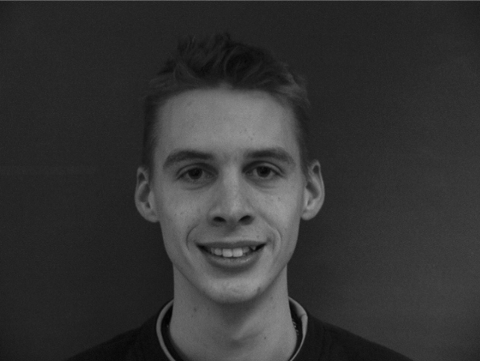Figure 2. Gray image

## 3.png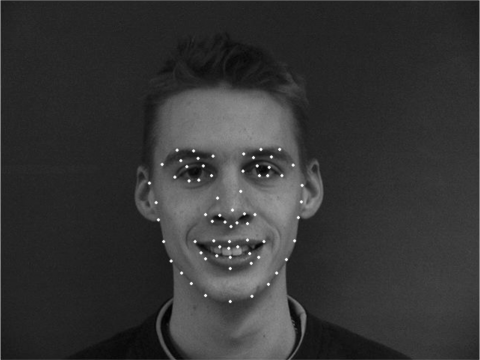Figure 3. Face landmark positioning

## 4.pngFigure 4. Subgraph division

## 5.png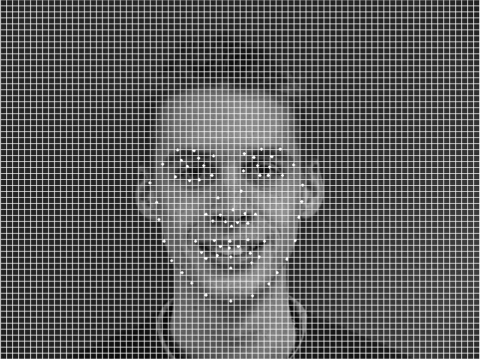Figure 5. Overlapping region

## 6.png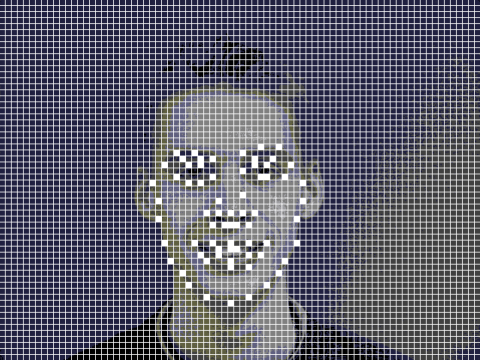Figure 6. Sensitive area (seed)

## 7.png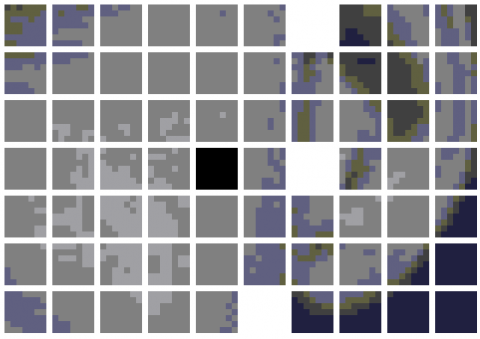Figure 7. Candidate areas for merging

## 8.png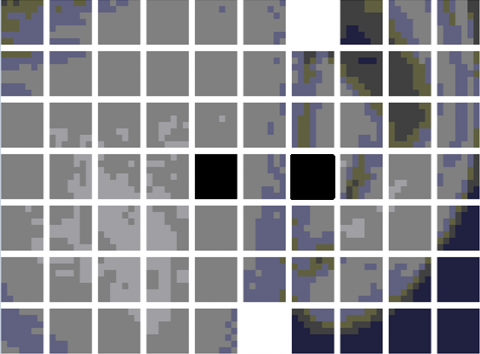Figure 8. Ideal areas for merging

## 9.png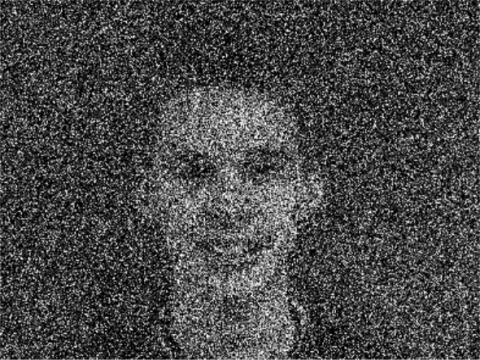Figure 9. Results of the LAP

## 10.png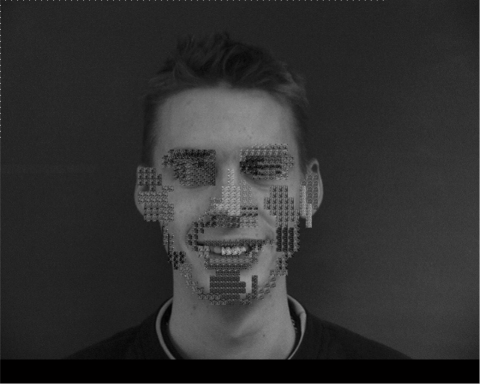Figure 10. Results of the DPLP

As shown in Figure 7, when a subgraph (black area) could be merged with multiple seeds (white area) in the DPLP, the merging result depends too much on the sequence of seed selection. To prevent the attacker from predicting the target face from landmark distribution, the Euclidean distance between each subgraph and each seed is adopted as the scoring function of the exponential mechanism to guide the selection of areas to be merged. Figure 8 shows the most reasonable merging method under ε2.

Figure 9 presents the results of the LAP algorithm, and Figure 10 displays the results of the DPLP under the same privacy budget. It can be observed that the DPLP only functions within the regional growth range with face landmarks as seeds, while the LAP acts on the entire image.

4.2 Results analysis

To verify the feasibility of our algorithm, some multi-face images were selected from IMM Face Database, Aberdeen Face Database, and LFW Face Database, and compiled into test sets. The experimental environment includes Intel® Core i9-9900K CPU @ 3.60 GHz, 32G memory, GTX 21080TI GPU, and Windows 10. During the experiments, the faces were recognized by TensorFlow + AlexNet CNN. The privacy budget ε was set to 0.5, 1, 1.5, 2, 2.5, and 3, in turn. The performance was measured by Precision, Recall, and F1-score. The experimental results are displayed in Figures 11-19.

## 11.png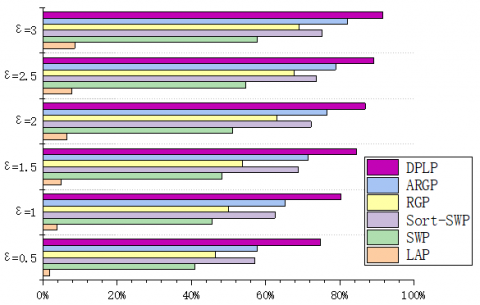Figure 11. Precision on IMM

## 12.png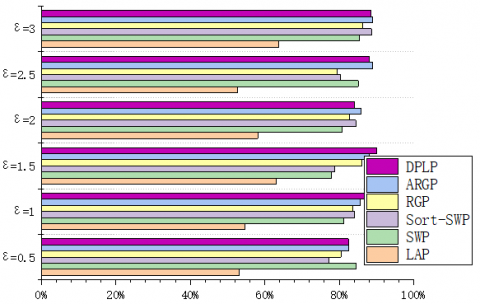Figure 12. Recall on IMM

## 13.pngFigure 13. F1-score on IMM

## 14.png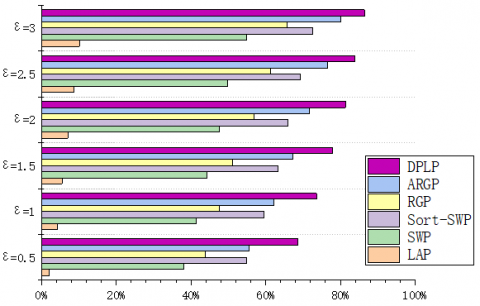Figure 14. Precision on Aberdeen

## 15.png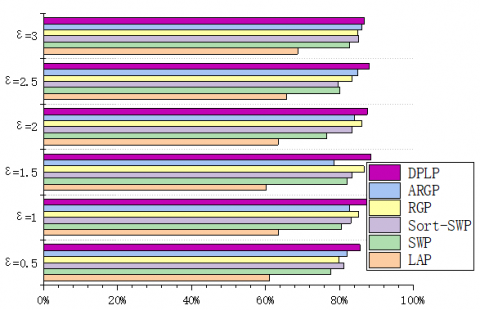Figure 15. Recall on Aberdeen

## 16.png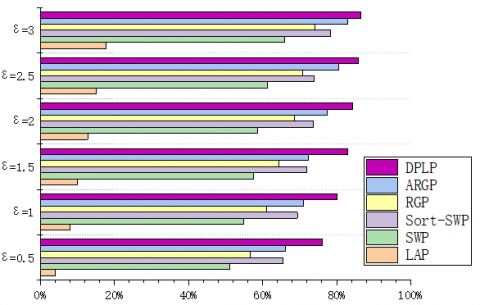Figure 16. F1-score on Aberdeen

## 17.png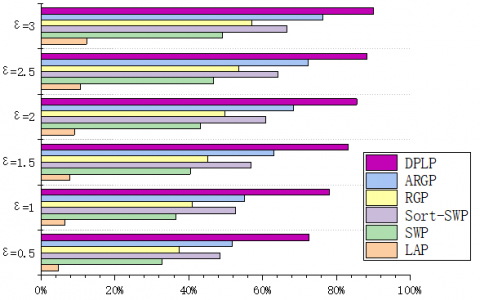Figure 17. Precision on LFW

## 18.png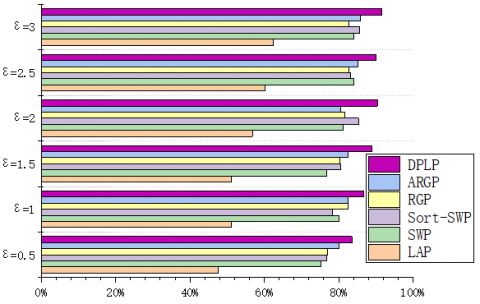Figure 18. Recall on LFW

## 19.png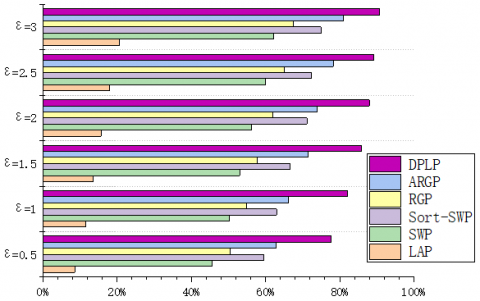Figure 19. F1-score on LFW

Under the same privacy budget (ε=0.5), the LAP performed the best on LFW (250*250) (Precision=4.7%), followed by Aberdeen (400*550) (Precision=2.1%), and worked the worst on IMM (480*640) (Precision=1.8%). This is because the total error of the LAP depends on the image size. For sliding window publication (SWP) algorithm, sort-SWP algorithm, region growing publication (RGP), and atypical RGP (ARGP) algorithm, the total error hinges on the regional growth results. Under the same privacy budget and Th, the results of regional growth depend on the complexity of the image. All four algorithms had the worst performance (Precision=32.7%, 48.6%, 37.5%, 51.8%) on LFW, due to the complex background of images in the database. Their performances on IMM (Precision=40.9%, 57.2%, 46.6%, 57.7%) and Aberdeen (Precision=38.2%, 54.9%, 43.8%, 55.5%) were similar, both were better than the performance on LFW. This is attributable to the fact that region merging is easier due to the pure color backgrounds of images in these two databases. In addition, the experimental results show that the error of the DPLP is independent of image size, but affected by the percentage of face area in the entire image. That is, the proportion of face area is negatively correlated with Precision, Recall, and F1-score. The result is consistent with the previous expectation.

5. Conclusions

To solve the problems in privacy protection of face images, this paper integrates face landmark positioning, regional growth, and Laplace mechanism in differential privacy to add noise to local sensitive areas in the original image, and thus realizes the non-global privacy protection of face images under the interactive framework. Through comparative experiments on several face databases (IMM, Aberdeen, and LFW), the proposed DPLP achieved better Precision, Recall, and F1-score than LAP, SWP, Sort-SWP, RGP, and ARGP. The results suggest that DPLP-based privacy protection of face images improves the availability of the protected image. Using DPLP, the protected areas in the original image only depend on landmark positions and growth rules. Thus, the noise error induced by privacy protection does not change with image size.

Notably, although the DPLP can effectively protect the face information in face images, the algorithm is unable to pinpoint the landmarks in multiple faces, if the original image contains more than one faces, and the face areas only account for a small portion of the image. In this case, some face information in the image may be leaked. To prevent the privacy leak, the future research will further improve the face image privacy protection framework for multi-face images.

Acknowledgment

The authors thank the project of the National Natural Science Foundation of China (Grant No.: 61672179), Natural Science Foundation of Heilongjiang Province (Grant No.: LH2021D022, 2019F004), and Fundamental Research Funds in Heilongjiang Provincial Education Department (Grant No.: 145109216).

References

 Liu, C., Yang, J., Zhao, W., Zhang, Y., Li, J., Mu, C. (2021). Face image publication based on differential privacy. Wireless Communications and Mobile Computing, (9): 1-20. https://doi.org/10.1155/2021/6680701

 Fung, B.C., Wang, K., Philip, S.Y. (2007). Anonymizing classification data for privacy preservation. IEEE Transactions on Knowledge and Data Engineering, 19(5): 711-725. https://doi.org/10.1109/TKDE.2007.1015

 Xiao, X., Tao, Y. (2006). Anatomy: Simple and effective privacy preservation. In Proceedings of the 32nd International Conference on Very Large Data Bases, pp. 139-150. https://doi.org/10.3390/met11020309

 Li, T., Li, N., Zhang, J., Molloy, I. (2010). Slicing: A new approach for privacy preserving data publishing. IEEE Transactions on Knowledge and Data Engineering, 24(3): 561-574. https://doi.org/10.1109/TKDE.2010.236

 Terrovitis, M., Liagouris, J., Mamoulis, N., Skiadopoulos, S. (2012). Privacy preservation by disassociation. arXiv preprint arXiv:1207.0135.

 Sweeney, L. (2002). k-anonymity: A model for protecting privacy. International Journal of Uncertainty, Fuzziness and Knowledge-Based Systems, 10(5): 557-570. https://doi.org/10.1142/S0218488502001648

 Dwork, C. (2006). Differential privacy. In International Colloquium on Automata, Languages, and Programming, pp. 1-12. https://doi.org/10.1007/11787006_1

 Dwork, C. (2008). Differential privacy: A survey of results. In International Conference on Theory and Applications of Models of Computation, pp. 1-19. https://doi.org/10.1007/978-3-540-79228-4_1

 Dwork, C. (2009). The differential privacy frontier. In Theory of Cryptography Conference, pp. 496-502.

 Dwork, C., Lei, J. (2009). Differential privacy and robust statistics. In Proceedings of the Forty-First Annual ACM Symposium on Theory of Computing, pp. 371-380. https://doi.org/10.1145/1536414.1536466

 Dwork, C. (2010). Differential privacy in new settings. In Proceedings of the twenty-first annual ACM-SIAM symposium on Discrete Algorithms, pp. 174-183. https://doi.org/10.1137/1.9781611973075.16

 Dwork, C. (2011). The promise of differential privacy a tutorial on algorithmic techniques. In 2011 IEEE 52nd Annual Symposium on Foundations of Computer Science, pp. 1-2.

 Dwork, C., McSherry, F., Nissim, K., Smith, A. (2006). Calibrating noise to sensitivity in private data analysis. In Theory of Cryptography Conference, pp. 265-284. https://doi.org/10.1007/11681878_14

 McSherry, F., Talwar, K. (2007). Mechanism design via differential privacy. In 48th Annual IEEE Symposium on Foundations of Computer Science (FOCS'07), pp. 94-103. https://doi.org/10.1109/FOCS.2007.66

 McSherry, F.D. (2009). Privacy integrated queries: an extensible platform for privacy-preserving data analysis. In Proceedings of the 2009 ACM SIGMOD International Conference on Management of data, pp. 19-30. https://doi.org/10.1145/1559845.1559850

 Roth, A., Roughgarden, T. (2010). Interactive privacy via the median mechanism. In Proceedings of the forty-second ACM symposium on Theory of Computing, pp. 765-774. https://doi.org/10.1145/1806689.1806794

 Hardt, M., Rothblum, G.N. (2010). A multiplicative weights mechanism for privacy-preserving data analysis. In 2010 IEEE 51st Annual Symposium on Foundations of Computer Science, pp. 61-70. https://doi.org/10.1109/FOCS.2010.85

 Gupta, A., Roth, A., Ullman, J. (2012). Iterative constructions and private data release. In Theory of Cryptography Conference, pp. 339-356. https://doi.org/10.1007/978-3-642-28914-9_19

 Fan, L., Xiong, L. (2012). Real-time aggregate monitoring with differential privacy. In Proceedings of the 21st ACM International Conference on Information and Knowledge Management, pp. 2169-2173. https://doi.org/10.1145/2396761.2398595

 Kellaris, G., Papadopoulos, S., Xiao, X., Papadias, D. (2014). Differentially private event sequences over infinite streams. Proceedings of the VLDB Endowment, 7(12): 1155-1166. https://doi.org/10.14778/2732977.2732989

 Xiao, X., Wang, G., Gehrke, J. (2010). Differential privacy via wavelet transforms. IEEE Transactions on Knowledge and Data Engineering, 23(8): 1200-1214. https://doi.org/10.1109/TKDE.2010.247

 Xu, J., Zhang, Z., Xiao, X., Yang, Y., Yu, G., Winslett, M. (2013). Differentially private histogram publication. The VLDB Journal, 22(6): 797-822. https://doi.org/10.1007/s00778-013-0309-y

 Li, C., Miklau, G., Hay, M., McGregor, A., Rastogi, V. (2015). The matrix mechanism: Optimizing linear counting queries under differential privacy. The VLDB Journal, 24(6): 757-781. https://doi.org/10.1007/s00778-015-0398-x

 Li, C., Hay, M., Miklau, G., Wang, Y. (2014). A data- and workload-aware algorithm for range queries under differential privacy. Proceedings of the VLDB Endowment, 7(5): 341-352. https://doi.org/10.14778/2732269.2732271

 Yang, G., Huang, T.S. (1994). Human face detection in a complex background. Pattern recognition, 27(1): 53-63. https://doi.org/10.1016/0031-3203(94)90017-5

 Meng, W.L., Mao, C.Z., Zhang, J., Wen, J., Wu, D.H. (2019). A fast recognition algorithm of online social network images based on deep learning. Traitement du Signal,  36(6): 575-580. https://doi.org/10.18280/ts.360613

 Feng, G.C., Yuen, P.C. (1998). Variance projection function and its application to eye detection for human face recognition. Pattern Recognition Letters, 19(9): 899-906. https://doi.org/10.1016/S0167-8655(98)00065-8

 Zhou, Z.H., Geng, X. (2004). Projection functions for eye detection. Pattern Recognition, 37(5): 1049-1056. https://doi.org/10.1016/j.patcog.2003.09.006

 Zhang, L., Lenders, P. (2000). Knowledge-based eye detection for human face recognition. In KES'2000. Fourth International Conference on Knowledge-Based Intelligent Engineering Systems and Allied Technologies. Proceedings (Cat. No. 00TH8516), (1): 117-120. https://doi.org/10.1109/KES.2000.885772

 Reisfeld, D., Yeshurun, Y. (1992). Robust detection of facial features by generalized symmetry. In International Conference on Pattern Recognition, pp. 117-117. https://doi.org/10.1109/ICPR.1992.201521

 Wu, H., Yokoyama, T., Pramadihanto, D., Yachida, M. (1996). Face and facial feature extraction from color image. In Proceedings of the Second International Conference on Automatic Face and Gesture Recognition, pp. 345-350. https://doi.org/10.1109/AFGR.1996.557289

 Yuille, A.L., Hallinan, P.W., Cohen, D.S. (1992). Feature extraction from faces using deformable templates. International Journal of Computer Vision, 8(2): 99-111. https://doi.org/10.1007/BF00127169

 Lanitis, A., Taylor, C.J., Cootes, T.F. (1995). A unified approach to coding and interpreting face images. In Proceedings of IEEE International Conference on Computer Vision, pp. 368-373. https://doi.org/10.1109/ICCV.1995.466919

 Cootes, T.F., Hill, A., Taylor, C.J., Haslam, J. (1993). The use of active shape models for locating structures in medical images. Image and Vision Computing, 12(6): 355-365. https://doi.org/10.1007/BFb0013779

 Dollár, P., Welinder, P., Perona, P. (2010). Cascaded pose regression. In 2010 IEEE Computer Society Conference on Computer Vision and Pattern Recognition, pp. 1078-1085. https://doi.org/10.1109/CVPR.2010.5540094

 LeCun, Y., Bottou, L., Bengio, Y., Haffner, P. (1998). Gradient-based learning applied to document recognition. Proceedings of the IEEE, 86(11): 2278-2324. https://doi.org/10.1109/5.726791

 Sun, Y., Wang, X., Tang, X. (2013). Deep convolutional network cascade for facial point detection. In Proceedings of the IEEE Conference on Computer Vision and Pattern Recognition, pp. 3476-3483.

 Zhang, Z., Luo, P., Loy, C.C., Tang, X. (2014). Facial landmark detection by deep multi-task learning. In European Conference on Computer Vision, pp. 94-108. https://doi.org/10.1007/978-3-319-10599-4_7

 Zhang, K., Zhang, Z., Li, Z., Qiao, Y. (2016). Joint face detection and alignment using multitask cascaded convolutional networks. IEEE Signal Processing Letters, 23(10): 1499-1503. https://doi.org/10.1109/LSP.2016.2603342

 Wu, Y., Hassner, T., Kim, K., Medioni, G., Natarajan, P. (2017). Facial landmark detection with tweaked convolutional neural networks. IEEE Transactions on Pattern Analysis and Machine Intelligence, 40(12): 3067-3074. https://doi.org/10.1109/TPAMI.2017.2787130

 Reddy, C., Reddy, U.S., Kishore, K. (2019). Facial emotion recognition using NLPCA and SVM. Traitement du Signal, 36(1): 13-22. https://doi.org/10.18280/ts.360102

 Liu, C., Yang, J., Wu, J. (2020). Web intrusion detection system combined with feature analysis and SVM optimization. EURASIP Journal on Wireless Communications and Networking, (1): 1-9. https://doi.org/10.1186/s13638-019-1591-1

 Yow, K.C., Cipolla, R. (1996). A probabilistic framework for perceptual grouping of features for human face detection. In Proceedings of the Second International Conference on Automatic Face and Gesture Recognition, pp. 16-21. https://doi.org/10.1109/AFGR.1996.557238

 Wiskott, L., Krüger, N., Kuiger, N., Von Der Malsburg, C. (1997). Face recognition by elastic bunch graph matching. IEEE Transactions on pattern analysis and machine intelligence, 19(7): 775-779. https://doi.org/10.1109/34.598235

 Feris, R.S., Gemmell, J., Toyama, K., Kruger, V. (2002). Hierarchical wavelet networks for facial feature localization. In Proceedings of Fifth IEEE International Conference on Automatic Face Gesture Recognition, pp. 125-130. https://doi.org/10.1109/AFGR.2002.1004143

 Liu, C., Yang, J., Zhao, W., Zhang, Y., Shi, C., Miao, F., Zhang, J. (2021). Differential privacy protection of face images based on region growing. Traitement du Signal, 38(5): 1385-1401. https://doi.org/10.18280/ts.380514